### Home > INT3 > Chapter Ch9 > Lesson 9.1.5 > Problem9-65

9-65.
1. Your calculator can function in both degrees and radians. See if you can determine how to put your calculator in radian mode and then how to switch it back to degree mode. On most scientific calculators, a small “DEG” or “RAD” shows on the screen to let you know in which mode you are working. Homework Help ✎

1. With your calculator in degree mode, calculate sin(60º). Then switch to radian mode and determine sin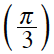. Did you get the same answer? Explain why your answers are the same or different.

2. What is sin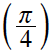? Which angles, measured in degrees, would have the same sine as sin?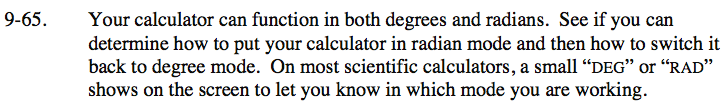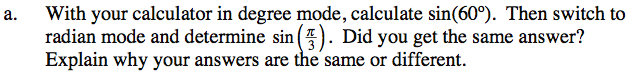$\text{What is }60\degree\text{ in radians? } \frac{\pi}{3}\text{ in degrees?}$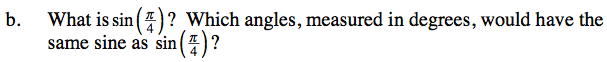45°, 135°, 405°, etc.